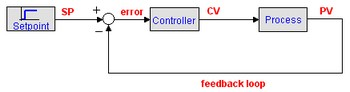﻿ 20-sim webhelp > Library > Signal > Control > PID Control > Setpoint and other Variables

# Setpoint and other Variables

A standard control loop is shown in the figure below. A controller tries to steer a process in a way that minimizes the difference between a given setpoint and the output of that process. In other words, the controller tries to get the process output as close as possible to the given setpoint.## Setpoint

The setpoint (SP) is where the process output should match. It can be a static value (e.g. the desired temperature of a room) or a varying value (e.g. the desired position of a robot-tip).

The setpoint is where you would like the measurement to be. Error is defined as the difference between set-point and measurement.

## Process

The process is the system that should be controlled. It is sometimes referred to as plant or system. The process should have has at least one impute to control its behavior and at least one output that is a measure of its behavior. Consider for example a gas heated house as process. The heater feed could be the process input and the measured room temperature the process output.

The process input is connected to the controller output or controller variable (CV). It is mostly the input for an actuator that can drive the process to a desired setpoint.

The process output is commonly known as process variable (PV), or measured variable (MV). Other names of the process output are system output and measurement.

## Error

The error is defined as the setpoint minus the process output. It is a measure for how much the process deviates from the desired setpoint, and thus how much the controller should respond to get the process back to the desired setpoint.

## Setpoint Weighting

For many controllers the controller input is equal to the error signal. Some controllers, however use specialized inputs for their proportional, integral and derivative parts (setpoint weighting). They have two inputs, one for the setpoint and one for the measured variable and are therefore also described as 2-DOF controllers.